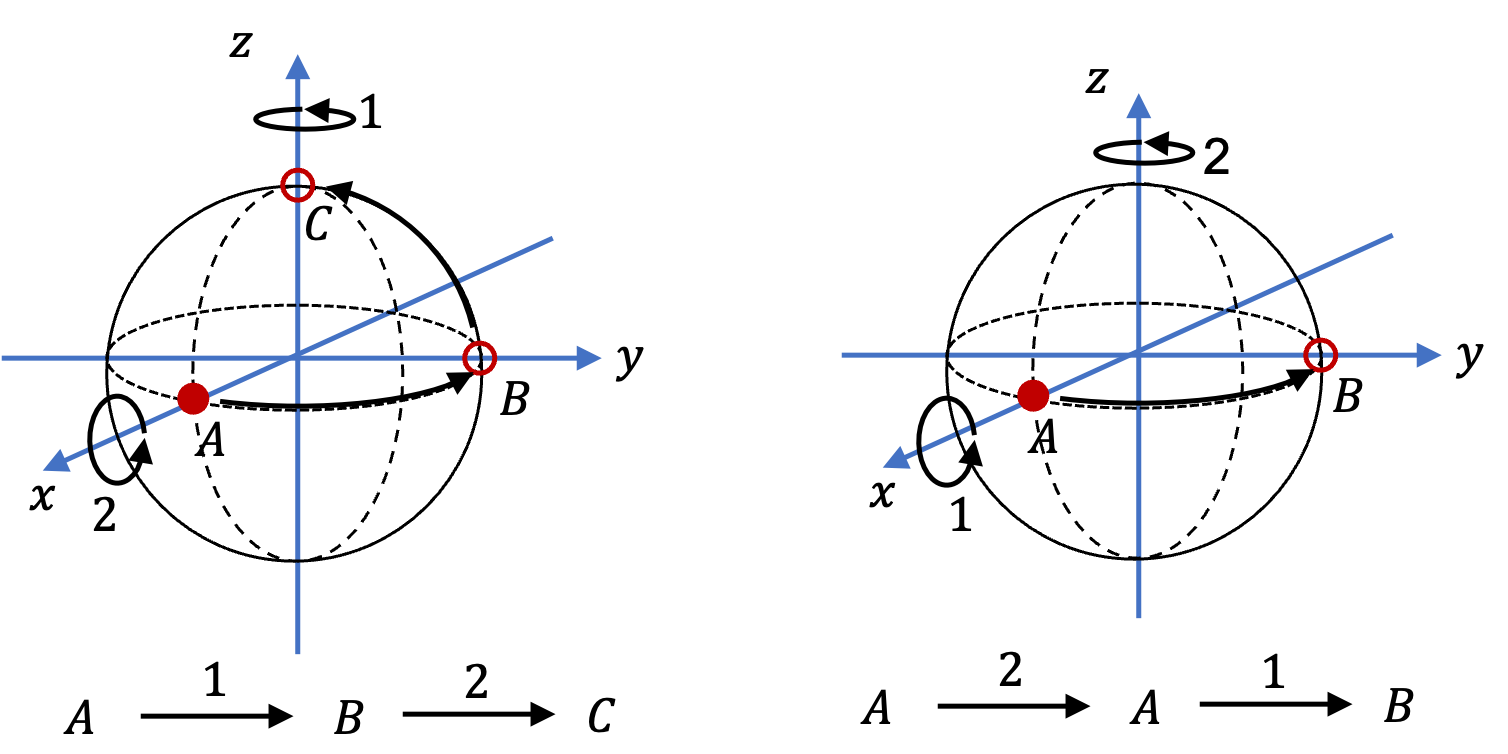## Section9.2Rotation Angle as a Vector

(Requires Calculus)

Could we include information about axis in the angle of rotation? Say, you rotate about $z$-axis by $\pi/2$ rad, then, could we write this as a vector $\frac{\pi}{2}\, \hat k\,\text{?}$ It turns out that we cannot do this. Let's illustrate this by rotating a point mass as in Figure 9.2.1 about $z$ and $x$ axes. The result of rotation about $z$-axis followed by $x$-axis is different than rotation about $x$-axis followed by $z$-axis. If we could write rotation angle as a vector, we will get an absurdity: $\vec \theta_1 + \vec \theta_2$ $\ne$ $\vec \theta_2 + \vec \theta_1 \text{.}$Figure 9.2.1. Left: Rotation of a point mass about $z$-axis followed by a rotation about $x$-axis moves the particle to point C. Right: A rotation $x$-axis followed by a rotation about $y$-axis moves the particle to point B.

Although, we cannot treat a rotated angle as a vector, the situation is different if angles are infinitesimal as shown in Figure 9.2.2.

Each angle can be states as $ds/R$ with movement of distance $ds$ on the arc. In the limit of infinitsimal displacement, they will become straight and the triangle of vectors will give net displacement that will be vector sum.

That is, the difference between a rotation about $d\theta_1$ about $z$-axis followed by $d\theta_2$ about $x$-axis and a rotation about $d\theta_2$ about $x$-axis followed by$d\theta_1$ about $z$-axis would be negligible so that we can treat these infinitesimal rotations as vectors with net rotation, $d\theta_1\,\hat k + d\theta_2\,\hat i.$
Hence, we can incorporate axis of rotation in analytic description of rotation by using infinitesimal rotation vectors with direction of vector being the axis of rotation and the angle of rotation being $d\theta\text{.}$ Thus, for an arbitrary axis $\hat u$ of rotation we will write